The GLMSELECT Procedure
 Criteria Used in Model Selection Methods

PROC GLMSELECT supports a variety of fit statistics that you can specify as criteria for the CHOOSE=, SELECT=, and STOP= options in the MODEL statement. The following statistics are available:

adjusted R-square statistic (Darlington 1968; Judge et al. 1985)

AIC

Akaike’s information criterion (Darlington 1968; Judge et al. 1985)

AICC

corrected Akaike’s information criterion (Hurvich and Tsai 1989)

BIC

Sawa Bayesian information criterion (Sawa 1978; Judge et al. 1985)

CP

Mallows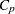statistic (Mallows 1973; Hocking 1976)

PRESS

predicted residual sum of squares statistic

SBC

Schwarz Bayesian information criterion (Schwarz 1978; Judge et al. 1985)

SL

significance level of thestatistic used to assess an effect’s contribution to the fit when it is added to or removed from a model

VALIDATE

average square error over the validation data

Table 42.7 provides formulas and definitions for the fit statistics.

Table 42.7 Formulas and Definitions for Model Fit Summary Statistics

Statistic

Definition or FormulaNumber of observationsNumber of parameters including the interceptEstimate of pure error variance from fitting the full model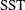Total sum of squares corrected for the mean for the
dependent variableError sum of squares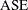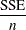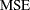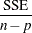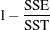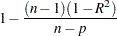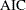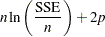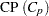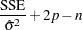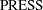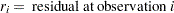and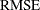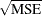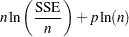### Changes in Formulas for AIC and AICC

The formulas used for the AIC and AICC statistics have been changed in SAS 9.2. However, the models selected at each step of the selection process and the final selected model are unchanged from the experimental download release of PROC GLMSELECT, even in the case where you specify AIC or AICC in the SELECT=, CHOOSE=, and STOP= options in the MODEL statement. The reason for making this change is to make the connection between the AIC statistic and the AICC statistic more transparent.

In the context of linear regression, several different versions of the formulas for AIC and AICC appear in the statistics literature. However, for a fixed number of observations, these different versions differ by additive and positive multiplicative constants. Because the model selected to yield a minimum of a criterion is not affected if the criterion is changed by additive and positive multiplicative constants, these changes in the formula for AIC and AICC do not affect the selection process.

The following section provides details about these changes. Formulas used in the experimental download release are denoted with a superscript ofand,andare defined in Table 42.7.

In the experimental download release of PROC GLMSELECT the following formulas are used for AIC (Darlington 1968; Judge et al. 1985) and AICC (Hurvich, Simonoff, and Tsai 1998):and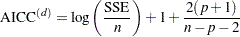The definitions of AIC and AICC used in this release are found in Hurvich and Tsai (1989). These formulas are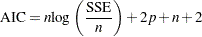andHurvich and Tsai (1989) show that the formula for AICC can also be written asThe relationships between the alternative forms of the formulas are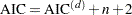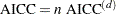Previous Page | Next Page | Top of Page